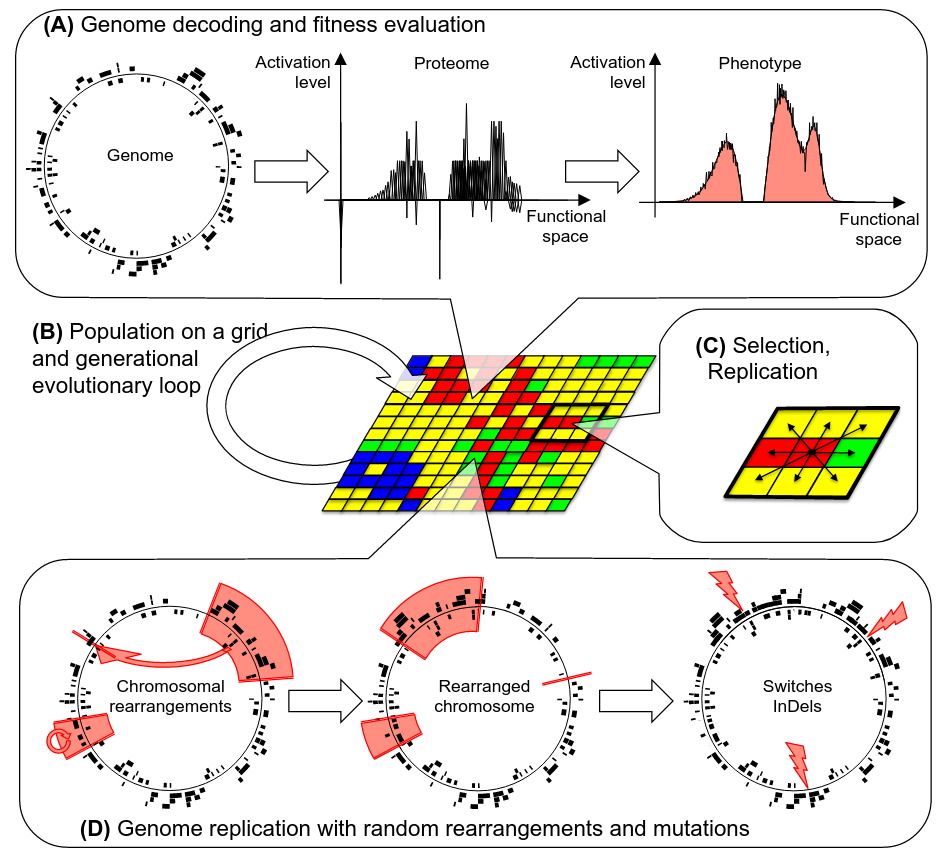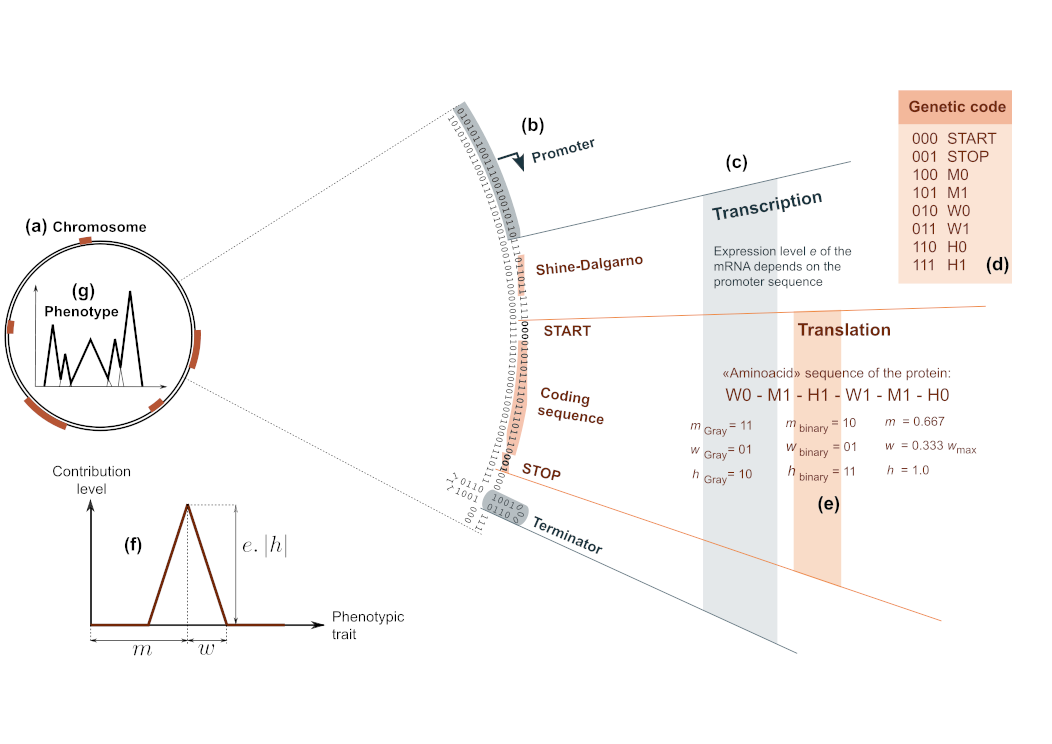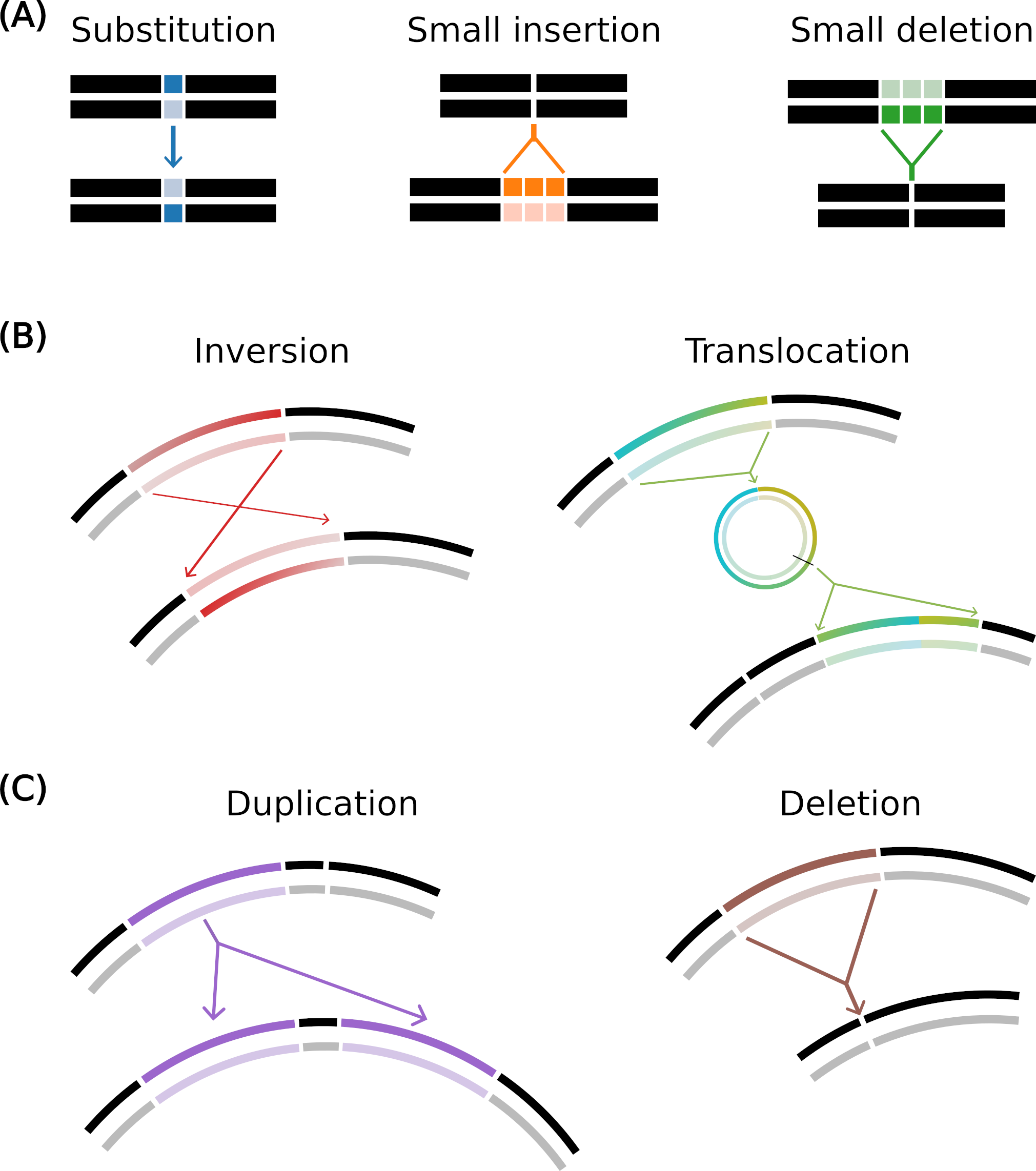# Model Description

## Introduction

Aevol is a forward-in-time evolutionary simulator that simulates the evolution of a population of haploid organisms through a process of variation and selection. The design of the model focuses on the realism of the genome structure and of the mutational process. Aevol can therefore be used to decipher the effect of chromosomal rearrangements on genome evolution, including their interactions with other types of mutational events.

In short, Aevol is made of three components (Fig. 1):

• A mapping that decodes the genomic sequence of an individual into a phenotype and computes the corresponding fitness value.
• A population of organisms, each owning a genome, hence its own phenotype and fitness. At each generation, the organisms compete to populate the next generation.
• A genome replication process during which genomes can undergo several kinds of mutational events, including chromosomal rearrangements and local mutations. The seven modelled types of mutation entail 3 local mutations: substitutions, small insertion, small deletion, 2 balanced rearrangements (which conserve the genome size): inversions and translocations, and 2 unbalanced rearrangements: duplications and deletions. This allows the user to study the effect of chromosomal rearrangements and their interaction with other kinds of events such as substitutions and InDels.Figure 1 - The Aevol model(A) Overview of the genotype-to-phenotype map. Note that the organism shown here is a real organism evolved within Aevol for 1,000,000 generations with a typical target. Hence, it contains many Open-Reading Frames (ORF) on both strands (left panel), many proteins (central panel) and it is well adapted to its environment (i.e. its phenotypic function — black curve on the right panel — is very close to the target function — light red plain curve). (B) Population on a grid is fully renewed every generation. (C) Example of a local selection process occurring with a 3 × 3 neighborhood (right). (D) Mutation operators include chromosomal rearrangements (duplications, deletions, translocations and inversions – here a translocation and an inversion are shown) and local mutations (substitutions and InDels).

The following describes in more details the three components of the model.

## The Genotype-to-Phenotype-to-Fitness map

### Genome representation.

Each artificial organism, similarly to prokaryotes, is asexual, haploid, and owns a single circular chromosome. The genome is encoded as a double-strand binary string containing a variable number of genes separated by non-coding sequences (Fig. 2a). Genes are delimited by predefined signaling sequences indicating transcription and translation. The number of proteins an organism owns thus depends on its signaling sequences, and can evolve through mutational events. These proteins are combined to produce the phenotype.Figure 2 - The Genotype-to-Phenotype-to-Fitness mapIn the model, each organism owns a circular double-strand binary chromosome (a) along which genes are delimited by predefined signaling sequences (b): promoters and terminators mark the boundaries of RNAs (c), within which coding sequences can in turn be identified between a Shine-Dalgarno-Start signal and a Stop codon. Each coding sequence is then translated into the primary sequence of a protein, using a predefined genetic code (d). This primary sequence is decoded as three real parameters called m, w and h (e). Proteins, phenotypes, and environments are represented similarly through mathematical functions that associate a level in [0, 1] to each abstract phenotypic trait in [0, 1]. For simplicity reasons, a protein’s contribution is a piecewise-linear function with a triangular shape: the m, w and h parameters correspond respectively to the position, half-width and height of the triangle (f). All the proteins encoded in the chromosome are then summed to compute the phenotype (g) that, once compared to the environmental target, can be used to compute the fitness of the individual.

Transcription starts at promoters, which are defined in the model as sequences that are close enough to an arbitrarily chosen consensus sequence ($$0101011001110010010110$$ in all simulations presented here, with at most $$d_{max} = 4$$ mismatches). The expression level $$e$$ of an mRNA is determined by the similarity between the actual promoter and the consensus sequence: $$e = 1 - \frac{d}{d_{max} + 1}$$ with $$d$$ the number of mismatches ($$d\leq d_{max}$$). This models the interaction of the RNA polymerase with the promoter, without additional regulation.

When a promoter is found, transcription proceeds until a terminator is reached. Terminators are defined as sequences that would form a stem-loop structure, as the $$\rho{}$$-independent bacterial terminators do. The stem size is here set to 4 and the loop size to 3.

The translation initiation signal is $$011011****000$$, corresponding to a Shine-Dalgarno-like sequence followed by a Start codon $$000$$. When this signal is found on an mRNA, the downstream Open-Reading-Frame (ORF) is read until the termination signal (the Stop codon $$001$$), is found. Each codon lying between the initiation and termination signals is translated into an abstract amino-acid’’ using an artificial genetic code, thus giving rise to the sequence of the protein (Fig. 2e). Transcribed sequences (mRNAs) can contain an arbitrary number of ORF, with some mRNAs possibly containing no ORF at all (non-coding mRNAs) and others possibly containing several ORFs (polycistronic mRNAs). Importantly, the relative fractions of non-coding, monocistronic and polycistronic mRNAs are not predefined but result from the evolutionary dynamics and are likely to be influenced by the evolutionary conditions.

### Protein function and phenotype computation.

We define an abstract continuous one-dimensional space $$\Omega{} = [0,1]$$ of phenotypic traits. Each protein is modeled as a mathematical function that associates a contribution level between -1.0 and 1.0 to a subset of phenotypic traits. The range of phenotypic traits to which a single protein can contribute is limited by $$2 \times W_{max}$$, where $$W_{max}$$ defines the maximum pleiotropy degree. Hence, increasing $$W_{max}$$ indirectly reduces the total number of proteins required to cover the whole phenotypic space. Similarly to the $$K$$ parameter of the classical $$NK$$-fitness landscape, increasing $$W_{max}$$ increases the level of pleiotropy and hence the ruggedness of the fitness landscape.

For simplicity, we use piecewise-linear functions with a symmetric, triangular shape to model protein effect (Fig. 2f). This way, only three parameters are needed to characterize the contribution of a given protein: the position $$m \in \Omega$$ of the triangle on the axis, its half-width $$w$$ ($$w \le W_{max}$$) and its height $$h \in [-1,1]$$. This means that this protein contributes to the phenotypic traits in $$[m-w, m+w]$$, with a maximal contribution $$h$$ for the traits closest to $$m$$. Thus, various types of proteins can co-exist, from highly efficient (high $$h$$) to poorly efficient (low $$h$$) and even inhibiting (negative $$h$$) and from highly specialized (low $$w$$) to versatile (high $$w$$).

In this framework, the primary sequence of a protein is interpreted in terms of three interlaced binary subsequences that will in turn be decoded as the values for the $$m$$, $$w$$ and $$h$$ parameters. For instance, the codon $$010$$ (resp. $$011$$) is translated into the single amino acid $$W0$$ (resp. $$W1$$), which means that it concatenates a bit $$0$$ (resp. $$1$$) to the code of $$w$$. Mutations in the coding sequences, including of course local mutations but also chromosomal rearrangements, can change these values and hence change the protein’s contribution to the phenotype.

The contribution of all the proteins encoded in the genotype of an organism are combined to get the final level for each phenotypic trait. This is done by first scaling all protein contributions by the transcription rate $$e$$ of the corresponding mRNA (see above), then by summing the mathematical functions of all the proteins, with bounds in $$0$$ and $$1$$. The resulting piecewise-linear function $$f_P: \Omega{} \rightarrow [0, 1]$$ is called the phenotype of the organism.

\medskip \noindent \textbf{Fitness computation.} In the model, fitness depends only on the difference between the levels of the phenotypic traits and target traits levels, which are defined by a user-defined mathematical function $$f_E: \Omega{} \rightarrow [0, 1]$$. This target function indicates the optimal level of each phenotypic trait in $$\Omega{}$$ and is called the environmental target. In usual Aevol experiments, $$f_E$$ is the sum of several Gaussian lobes with different standard deviation, maximal height and centers. It can be stable over evolutionary time, or change stochastically.

The difference between $$f_P$$ and $$f_E$$ is defined as $$\Delta:=\int_\Omega{}|f_E(x) - f_P(x)| dx,,, \forall x\in\Omega$$ and is called the metabolic error’’. It is used to measure adaptation penalizing both the under-realization and the over-realization of phenotypic traits. Given the metabolic error of an individual, its fitness $$f$$ is given by $$f:=\exp(-k \Delta)$$ with $$k$$ a fixed parameter regulating the selection strength (the higher $$k$$, the larger the effect of metabolic error variations on the fitness values).

## Population model and selection process.

The population is modelled as a toroidal grid with one individual per grid cell. At each generation, the fitness of each individual is computed, and the individuals compete to populate each cell of the grid at the next generation. This competition can be fully local (the 9 individuals in the neighborhood of a given cell competing to populate it at the next generation, Fig. 1C) or encompass a larger subpopulation. If the selection scope encompasses the whole population, all individuals compete for all grid cells. Importantly, the more local the selection scope, the more the population model diverges from the panmictic Wright-Fisher model as local selection increases the effective population size $$N_e$$ for a given census population size.

Given a selection scope, the individuals in the neighborhood $$\mathcal{N}$$ of a given grid-cell compete through a fitness-proportionate’’ selection scheme: the probability $$p_j$$, for an individual $$j$$ with fitness $$f_j$$ to populate the focal grid-cell at the next generation is given by $$p_j=f_j/\sum_{i\in \mathcal{N}} f_i$$.

## Genetic operators

During their replication, genomes can undergo sequence variations (Fig. 1D). An important feature of the model is that, given the Genotype-to-Phenotype map, any genome sequence can be decoded into a phenotype (although possibly with no trait activated if there is no ORF on the sequence). This allows to implement – and test – any kind of mutational process. In the classical usage of the simulator, seven different kinds of mutations are modelled (depicted on Fig. 3). Three mutations are local (substitutions and small insertions or deletions), and four are chromosomal rearrangements, either balanced (with no change in genome size): translocations and inversions, or unbalanced: duplications and deletions.Figure 3 - Mutation operators in Aevol(A) Local mutations: substitution (one base pair is mutated to another), small insertion and small deletion (a few base pairs are inserted or deleted). (B) Balanced chromosomal rearrangements: inversion (two points are drawn and the segment in between is rotated) and translocation (a segment is excised, circularized, re-cut and inserted elsewhere in the genome). (C) Unbalanced chromosomal rearrangements: duplication (copy-paste of a segment in the genome) and deletion (suppression of a segment of the genome).

Local mutations happen at a position uniformly drawn on the genome. Substitutions change a single nucleotide. InDels insert (or delete) a small sequence of random length – and random composition for insertions. The length of the sequence is drawn uniformly between 1 and a maximum value (6 by default). Notably, InDels occurring within an ORF can shift the reading frame or simply add/remove codons, resulting in very different evolutionary outcomes.

Chromosomal rearrangement breakpoints are uniformly drawn on the chromosome, the number of breakpoints depending on the type of rearrangement (Fig. 3). Hence, chromosomal rearrangements can be of any size between 1 and the total genome size, allowing to investigate the effect of small structural variants that are indeed observed in vivo.

The rates $$\mu_{t}$$ at which each type $$t$$ of genetic mutation occur are defined as a per-base, per-replication probability. This means that the number of spontaneous events is linearly dependent on the length of the genome. However, its fixation probability depends on its phenotypic effect (for instance, a mutation affecting exclusively an untranscribed region is likely to be neutral). Hence, the distribution of fitness effects (DFE) of any kind of mutation is not predefined but depends on the intertwining of its effect on the sequence, and of the genome structure. For example, the fraction of coding sequences or the spatial distribution of the genes along the chromosome change the probability of a given mutation to alter the phenotype, and the fitness, an effect that is especially important for chromosomal rearrangements. Having an emergent DFE instead of a predefined one enables investigating the complex direct and indirect effects of chromosomal rearrangements on the evolutionary dynamics.